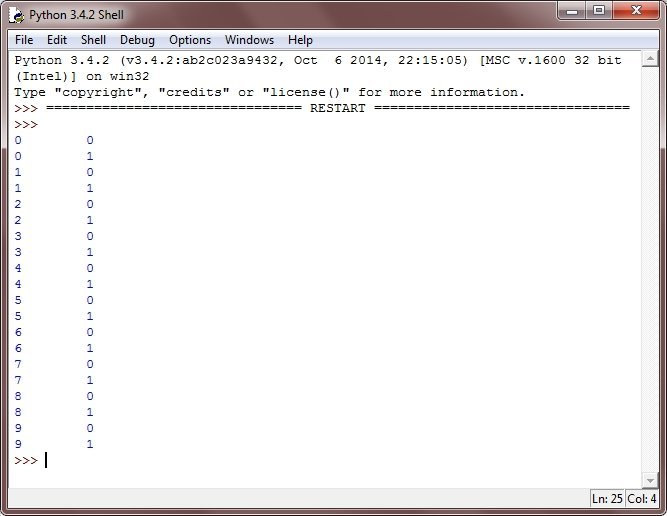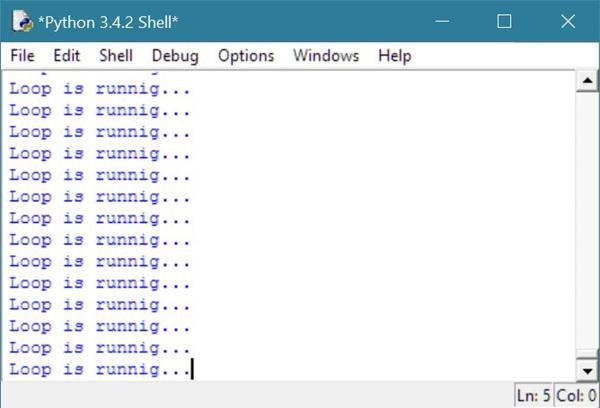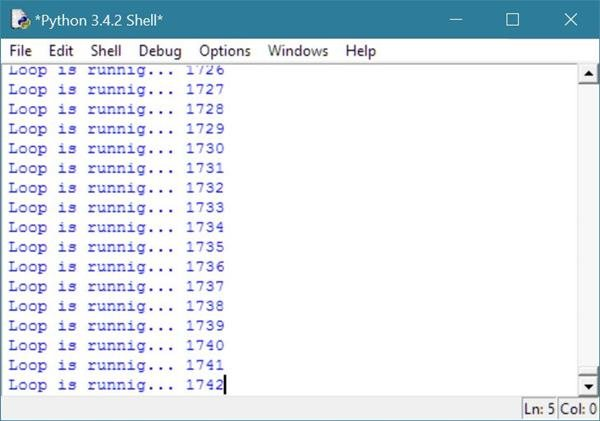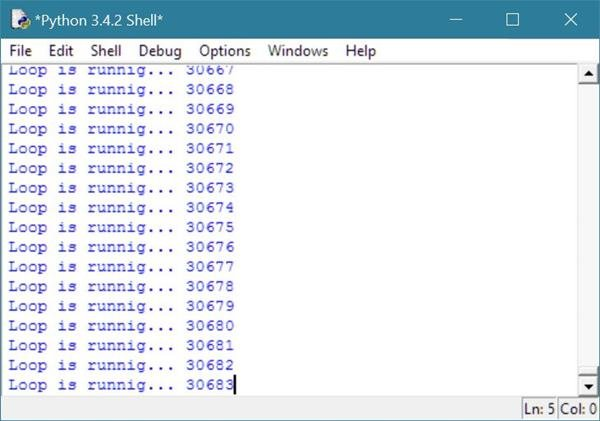# Python Loops

Loops in Python are used to control your program. Loops are basically used to execute a block of code several number of times accordingly. There are the following types of loops available in Python:

You will learn about for and while loop in separate tutorial.

## Python Loop Example Program

Here is an example illustrating loops in python:

```# Python Loops - Example Program

while True:
print("Enter 'x' for exit.")
val = input("Enter any number: ")
if val == 'x':
break
else:
num = int(val)
nrow = int(input("Upto how many rows: "))
for i in range(0, nrow):
for j in range(0, i+1):
print(num,end=" ")
print()```

Here is the sample run of the above python program:As you can see from the above python program, both for and while loops are used. Now let's take a look at nested loop in python.

Let's take another example of number guessing program using loop in python.

```# Python Loop Example Program
# Number guessing program in python
import random
random_number = random.randrange(11);
guess_number = int(input("Guess a number (under 11):"));
total_try = 1;
while(guess_number != random_number):
if(guess_number > random_number):
print("decrease the number..");
guess_number = int(input("Guess a number (under 11):"));
total_try += 1;
continue;
else:
print("increase the number..");
guess_number = int(input("Guess a number (under 11):"));
total_try += 1;
continue;
print("Wu hu!, you successfully guessed it!");
print("The number was",random_number);
print("You took only",total_try,"tries to guess it.");```

Here is the sample output produced by the above loop example program in python. Above example generate a random number while is less than 11, that is from 0 to 10, and ask from the user to guess that number. And the same time, above example also count the total number of try that have been done by the user to guess the correct number. Let's see the screenshot of some sample runs of the above loop program of python.Now start guessing the number as shown in following output:## Python nested Loop

You are free to use one loop inside another loop in python. Here is the general form to use nested for loop in python:

```for iterating-var in sequence:
for iterating-var in sequence:
statement(s)
statement(s)```

Here is the syntax to use nested while loop in python:

```while expression:
while expression:
statement(s)
statement(s)```

Here is an example, demonstrates the concept and use of nested loop in python:

```# Python Loops - Python nested Loop - Example Program

for i in range(10):
for j in range(2):
print(i, "\t", j)```

Here is the output produced by the above Python program:## Python Infinite Loop

Infinite loops in python are the loops that goes to run forever.

You can also say that infinite loop is the loop that never ends.

Let's take an example on infinite loop in python:

```# Python Infinite Loop Example
val = 1;
while(val != 0):
print("Loop is runnig...");
print("Loop end.");```

If you run the above infinite loop example code of python, then you will never see Loop end. on the output, rather you will continuously see the message, Loop is running.... Here is the sample output of the above example code of infinite loop in python:If you close the window, then below is the warning pop-up will be shown on your desktop, asking that, do you really want to exit the window, as infinite loop is running continuously. Here is the exact output you will see after closing the window where infinite loop is running.Let's modify the above infinite loop example to print the times that the loop is run as shown below.

```# Python Infinite Loop Example
val = 1;
times = 0;
while(val != 0):
print("Loop is runnig...",times);
times = times+1;
print("Loop end.");```

Below is the sample output of the above example of infinite loop.Here is the screenshot of the output taken after few time.### More Examples

Here are list of some more examples uses loops in python:

Tools
Calculator